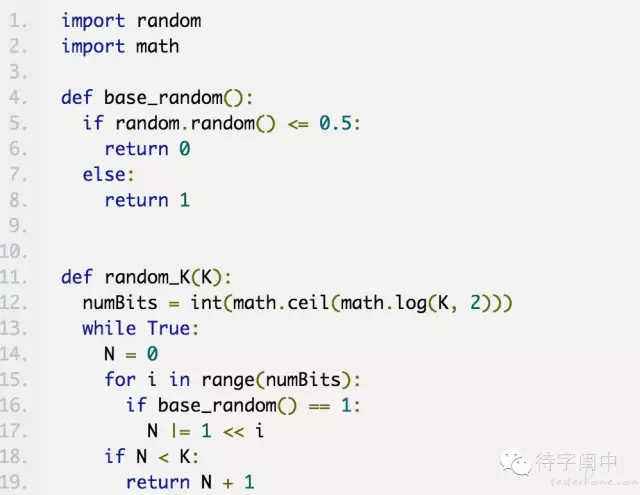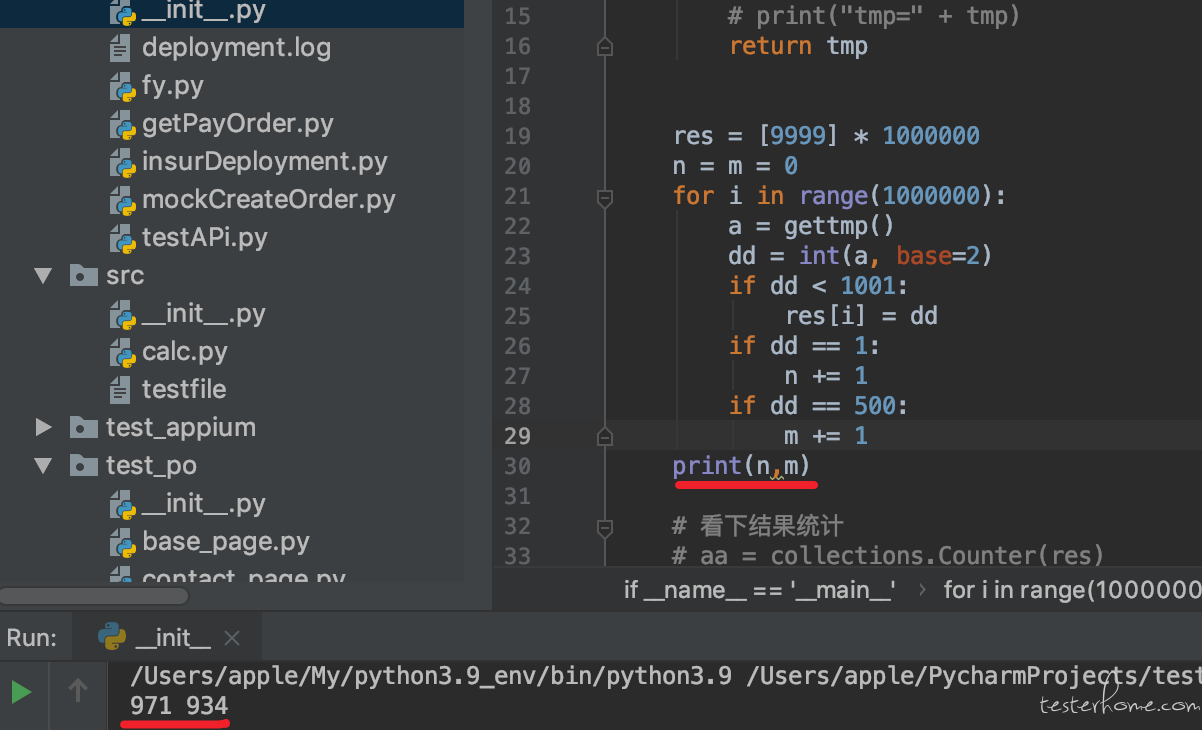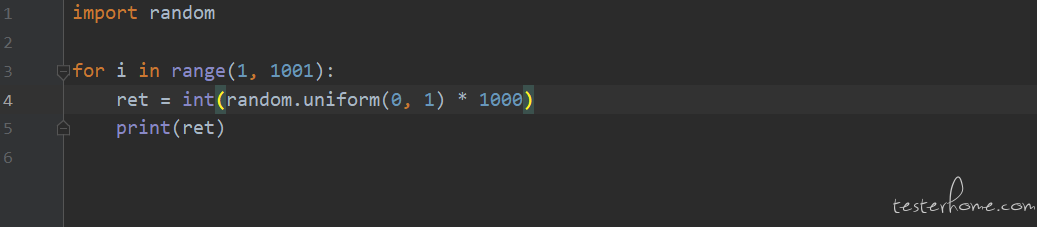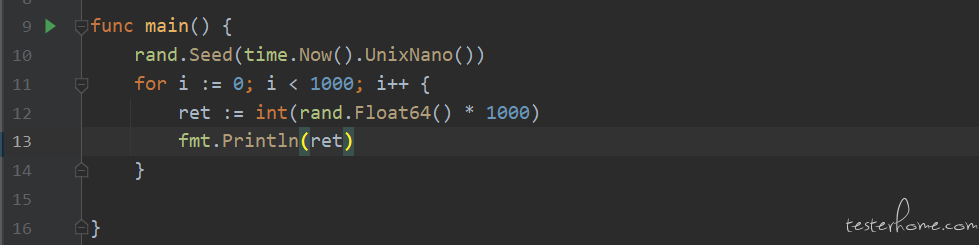# 刷题去WLB965 昨晚虾皮一面面试官出的一道题：利用随机生成（0，1）方法随机生成 0~1000 的数

def randomunm():
re=0
for i in range(0,1001):
re=random.randint(0,1)+re
return re

Math.ceil(random.nextDouble()*1000) 就行了吧。。。

#6 · 2021年09月10日 Author

• 生成 n 位随机浮点数 f
• 然后浮点转成字符串
• 字符串取 hash 值，然后再取绝对值
• 最后除 1000，取余 ``` abs(hash(str(f))) % 1000 ```
#13 · 2021年09月10日 Author

15楼 已删除

1000 = 512 + 256 + 128 + 64 + 32 + 8

``````# python
import random

a = [256, 128, 64, 32, 16, 8, 4, 2, 1]
b = []
for i in range(9+8+7+6+5+4):
b.append(random.Random().randint(0, 1))
data = [b[0:9], b[9:17], b[17:24], b[24:30], b[30:35], b[35:]]

def cal(data: list):
sum = 0
for j_data in data:
for i, value in enumerate(j_data):
sum += a[8-i]*value
return sum
print(data)
print(cal(data))
``````

[[1, 0, 1, 1, 1, 0, 1, 1, 0], [0, 0, 1, 1, 0, 0, 0, 0], [0, 1, 0, 1, 0, 1, 0], [1, 0, 1, 1, 1, 0], [0, 0, 0, 0, 0], [1, 1, 0, 1]]
315

“执行一万次，发现值分布均在 400-599 之间” 是不是我理解有问题。值不可能都分布在 400-500 之间吧，求指导

1、`随机生成 (0, 1)`，不知道是指只会随机生成 0 或者 1，还是生成 0-1 这个范围内的数字。
2、`生成 0~1000 的数`，不知道 0-1000 是范围还是啥。

rand 每次结果是独立的，把 10 次 rand 取值通过进制转换为 0-1024 的一个随机数，其中每一个概率都是一样的。去掉大于 1000 部分的就是 0-1000 的随机数了。

``````def rand():
return random.randint(0,1)

def gettmp():
tmp = ''
# 没次结果都是独立的，连续10次可以视为0-1024内均匀随机
for _ in range(10):
tmp +=str(rand())
return tmp
res = *1000000
for i in range(1000000):
dd = int(gettmp(),base=2)
if dd<1001:
res[i] = dd
# 看下结果统计
aa = collections.Counter(res)
# 去除掉9999（被拒绝掉的大于1000的部分）
aa.pop(9999)
# 出现最多的次数
print(max(aa.values())) #1077
# 出现最少的次数
print(min(aa.values())) #894
# 元素个数
print(len(aa))  #1001  [0-1000]

``````## 代码实现

``````import random
from collections import Counter

def get_str() -> str:
random_str = [str(random.randint(0, 1)) for _ in range(40)]
return "".join(random_str)

def get_hash(s) -> int:
return abs(hash(s))

def get_random() -> int:
s: str = get_str()
h: int = get_hash(s)
return h % 1001

def main():
res = []
for _ in range(10000):
r = get_random()
res.append(r)
c = Counter(res)
# 所有结果，最多只有1000个
count_all = c.most_common(1000)
# 出现次数最多的前10个
print(count_all[:10])
# 出现结果最少的10个
print(count_all[-10:])

d = dict(c)
# 1000出现的次数
print(d.get(1000))
# 500出现的次数
print(d.get(500))
# 0出现的次数
print(d.get(0))

if __name__ == '__main__':
main()
``````

## 运行结果

``````[(109, 23), (387, 21), (696, 20), (867, 20), (900, 19), (354, 19), (625, 19), (444, 19), (563, 18), (70, 18)]
[(738, 3), (77, 3), (603, 3), (800, 3), (106, 3), (780, 2), (228, 2), (22, 2), (778, 2), (107, 2)]
13
16
6
``````

``````import collections
import random

# 这是题目所给的rand1函数
def rand1():
return random.randint(0, 1)

# 实现1-4的随机函数
def rand4():
number1 = rand1() + 1
number2 = rand1()
while number2 == 1:
number2 = 2
return number1 + number2

# 根据rand4生成1-8的随机函数
def rand8():
number1 = rand4()
number2 = rand1()
while number2 == 1:
number2 = 4
return number1 + number2

# 根据rand8生成1-16的随机函数
def rand16():
"""
省略
"""

# 根据rand16生成1-32的随机函数
def rand32():
"""
省略
"""

"""

"""

# 根据rand512(),先拒绝采样，然后处理
def rand1000():
number1 = rand512()
while number1 > 500:
number1 = rand512()
number2 = rand1()
while number2 == 1:
number2 = 500
return number1 + number2

# 测试函数
ans = []
tar = 0
for _ in range(100000):
ans.append(rand32())
tar += 1
print(tar)
``````
27楼 已删除1111100000（992）跟 0000011111（31）不是一样的~

``````import random

def randomOutput():
out = ""
for _ in range(10):
a = random.choice([0, 1])
out += str(a)
if int(out, 2) > 1000:
return randomOutput()
else:
return int(out, 2)

b = []
for i in range(1000):
b.append(randomOutput())
``````

``````import random

data = []

def get_data():
while True:
for i in range(10):
data.append(str(random.randint(0, 1)))
data1 = int(''.join(data), 2)
if data1 <= 1000:
return data1
else:
data.clear()

``````